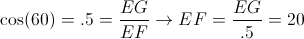# High School Math : Lines

## Example Questions

← Previous 1

### Example Question #1 : How To Find An Angle Of A Line

Linesandare parallel. Which angle is congruent to angle?Explanation:

When two lines are parallel, corresponding angles are congruent. Because we don't know if linesand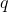are parallel, we can't make any conclusions about angles 2 and 3.

With the given information, we can ignore line, as it has no relation to angle. This leaves angles 1 and 4 as possible answers. Angle 4 will be congruent to angle, while angle 1 will supplement angle.

### Example Question #1 : Plane Geometry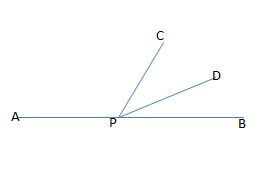Figure not drawn to scale.

In the figure above, APB forms a straight line. If the measure of angle APC is eighty-one degrees larger than the measure of angle DPB, and the measures of angles CPD and DPB are equal, then what is the measure, in degrees, of angle CPB?

50

40

66

114

33

66

Explanation:

Let x equal the measure of angle DPB. Because the measure of angle APC is eighty-one degrees larger than the measure of DPB, we can represent this angle's measure as x + 81. Also, because the measure of angle CPD is equal to the measure of angle DPB, we can represent the measure of CPD as x.

Since APB is a straight line, the sum of the measures of angles DPB, APC, and CPD must all equal 180; therefore, we can write the following equation to find x:

x + (x + 81) + x = 180

Simplify by collecting the x terms.

3x + 81 = 180

Subtract 81 from both sides.

3x = 99

Divide by 3.

x = 33.

This means that the measures of angles DPB and CPD are both equal to 33 degrees. The original question asks us to find the measure of angle CPB, which is equal to the sum of the measures of angles DPB and CPD.

measure of CPB = 33 + 33 = 66.

### Example Question #1 : Intersecting Lines And Angles

One-half of the measure of the supplement of angle ABC is equal to the twice the measure of angle ABC. What is the measure, in degrees, of the complement of angle ABC?

90

72

36

18

54

54

Explanation:

Let x equal the measure of angle ABC, let y equal the measure of the supplement of angle ABC, and let z equal the measure of the complement of angle ABC.

Because x and y are supplements, the sum of their measures must equal 180. In other words, x + y = 180.

We are told that one-half of the measure of the supplement is equal to twice the measure of ABC. We could write this equation as follows:

(1/2)y = 2x.

Because x + y = 180, we can solve for y in terms of x by subtracting x from both sides. In other words, y = 180 – x. Next, we can substitute this value into the equation (1/2)y = 2x and then solve for x.

(1/2)(180-x) = 2x.

Multiply both sides by 2 to get rid of the fraction.

(180 – x) = 4x.

180 = 5x.

Divide both sides by 5.

x = 36.

The measure of angle ABC is 36 degrees. However, the original question asks us to find the measure of the complement of ABC, which we denoted previously as z. Because the sum of the measure of an angle and the measure of its complement equals 90, we can write the following equation:

x + z = 90.

Now, we can substitute 36 as the value of x and then solve for z.

36 + z = 90.

Subtract 36 from both sides.

z = 54.

### Example Question #1 : Plane Geometry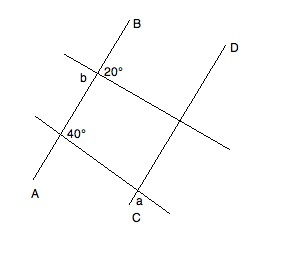In the diagram, AB || CD. What is the value of a+b?

60°

160°

140°

80°

160°

Explanation:

Refer to the following diagram while reading the explanation: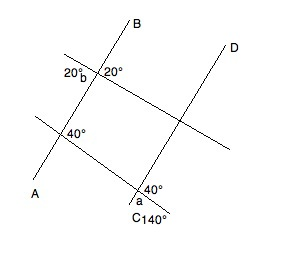We know that angle b has to be equal to its vertical angle (the angle directly "across" the intersection).  Therefore, it is 20°.

Furthermore, given the properties of parallel lines, we know that the supplementary angle to a must be 40°.  Based on the rule for supplements, we know that a + 40° = 180°.  Solving for a, we get a = 140°.

Therefore, a + b = 140° + 20° = 160°

### Example Question #4 : Lines

In rectangle ABCD, both diagonals are drawn and intersect at point E.

Let the measure of angle AEB equal x degrees.

Let the measure of angle BEC equal y degrees.

Let the measure of angle CED equal z degrees.

Find the measure of angle AED in terms of x, y, and/or z.

180 – y

360 – x + y + z

180 – 1/2(x + z)

180 – (x + y + z)

180 – 2(x + z)

180 – 1/2(x + z)

Explanation:

Intersecting lines create two pairs of vertical angles which are congruent. Therefore, we can deduce that y = measure of angle AED.

Furthermore, intersecting lines create adjacent angles that are supplementary (sum to 180 degrees). Therefore, we can deduce that x + y + z + (measure of angle AED) = 360.

Substituting the first equation into the second equation, we get

x + (measure of angle AED) + z + (measure of angle AED) = 360

2(measure of angle AED) + x + z = 360

2(measure of angle AED) = 360 – (x + z)

Divide by two and get:

measure of angle AED = 180 – 1/2(x + z)

### Example Question #1 : How To Find An Angle Of A Line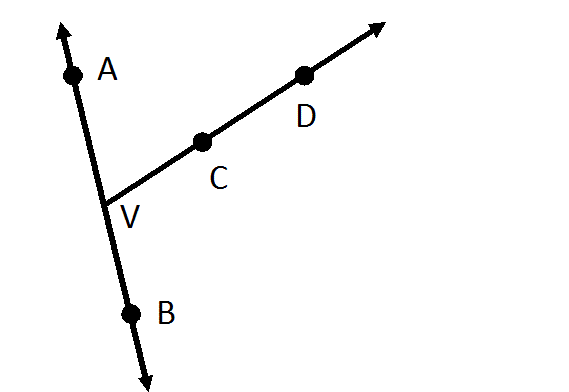Give another name for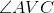.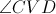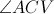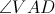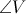Explanation:

Since the angle is called, it has vertex- the middle letter is always the vertex - and it is the union of rays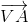and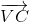. Another name foris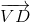, sinceis also on that ray, so the angle can be said to be the union ofand; this makes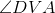a valid name for the angle.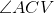andare not valid, since the middle letter is not vertex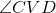is not valid, sinceandare on the same side of the angle.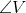is not valid; an angle can be named using only its vertex only if it is the only angle in the diagram with that vertex, and that is not the case here.

### Example Question #3 : How To Find An Angle Of A Line

Linesandare parallel. Which of the following pairs of angles are supplementary?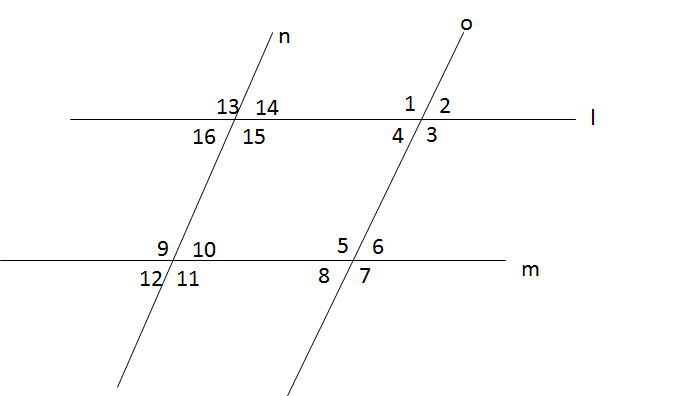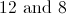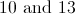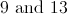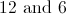Explanation:

Coresponding angles can be found when a line crosses two parallel lines. Angles 10 and 14 are equal, because corresponding angles are equal. Angles 14 and 13 are supplementary because together they form a straight line. If angles 10 and 14 are equal, then angles 10 and 13 must be supplementary as well.

### Example Question #6 : Lines

Two pairs of parallel lines intersect: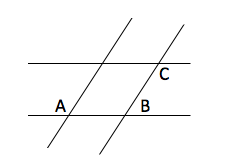If A = 135o, what is 2*|B-C| = ?

150°

160°

140°

180°

170°

180°

Explanation:

By properties of parallel lines A+B = 180o, B = 45o, C = A = 135o, so 2*|B-C| = 2* |45-135| = 180o

### Example Question #1 : How To Find An Angle Of A Line

Two angles are supplementary and have a ratio of 1:4.  What is the size of the smaller angle?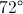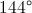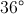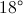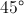Explanation:

Since the angles are supplementary, their sum is 180 degrees.  Because they are in a ratio of 1:4, the following expression could be written: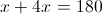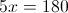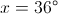### Example Question #1 : Geometry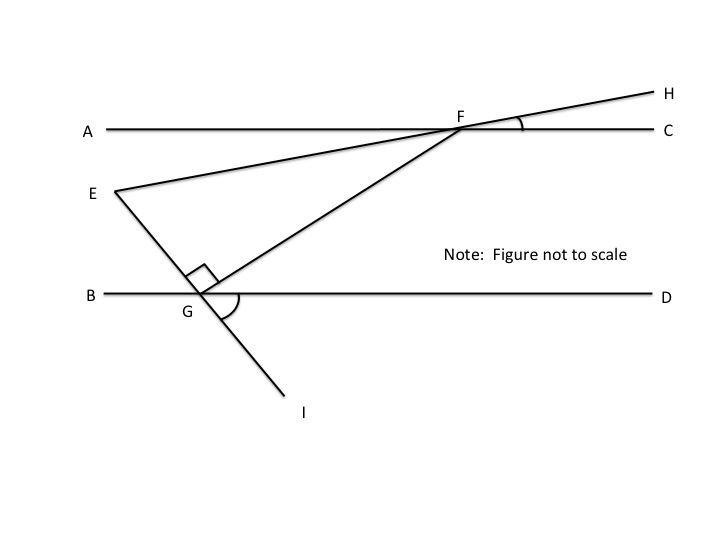Lines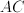and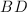are parallel.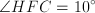,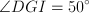,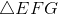is a right triangle, and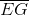has a length of 10.  What is the length of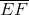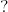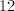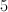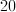Not enough information.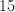Since we know opposite angles are equal, it follows that angle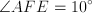and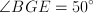.
Imagine a parallel line passing through point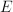.  The imaginary line would make opposite angles with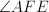&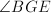, the sum of which would equal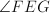.  Therefore,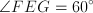.